3 Trapezoid Calculators
Scroll Down for instructions and definitions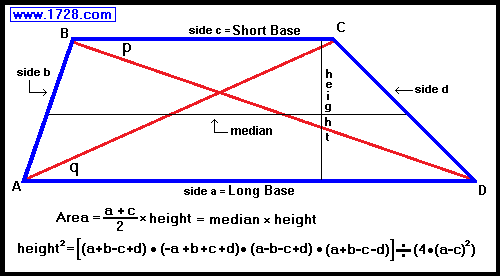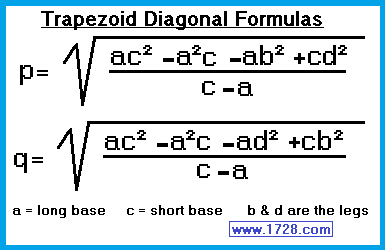Trapezoid area = ((sum of the bases) ÷ 2) • height
Lines BC and AD are parallel and are called bases.
Lines AB and DC are the non-parallel sides and are called legs.
Lines AC (or q) and BD (or p) are called diagonals
The line perpendicular to lines AD & BC is called the height or altitude.
The line parallel to lines AD & BC, is at the midpoints of lines AB and DC and is called the median or the midsegment.
The length of the median = (Line AD + Line BC) ÷ 2
Trapezoids have 2 pairs of adjacent angles (A & B) and (B & C) that are supplementary (add to 180°).

To use this calculator, you need the lengths of all 4 trapezoid sides.

 To use this calculator, you needboth base lengths and the area. To use this calculator, you need both base lengths and the height.
 * * * * * * * * * E x a m p l e * * * * * * * * * A trapezoid has bases that are 30 and 55 centimeters in length and the non-parallel sides (or legs) are 15 and 20 centimeters. What is the area of the trapezoid? Going by the diagram, we shall label the 4 sides as: a = 55     b = 15     c = 30     d = 20 Before we can use the area formula, we first have to determine the height of the trapezoid. (height)2 = (a+b-c+d) • (-a+b+c+d) • (a-b-c+d) • (a+b-c-d) ÷ (4 • (a -c)2) (height)2 = (55+15-30+20) • (-55+15+30+20) • (55-15-30+20) • (55+15-30-20) ÷ (4 • (55 -30)2) (height)2 = (60) • (10) • (30) • (20) ÷ (4 • (25)2) (height)2 = 360,000 ÷ 2,500 (height)2 = 144 height = 12 cm Now to use the area formula: trapezoid area = ((sum of the bases) ÷ 2) • height trapezoid area = ((55 + 30) ÷ 2) • 12 trapezoid area = 510 cm² To see how to calculate trapezoid area without using formulas, click here. * * * * * * * * * Trapezoids * * * * * * * * *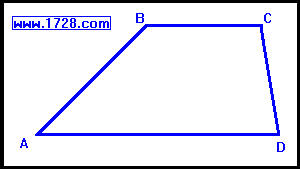ALL TRAPEZOIDS have the following properties:1) ONE pair of opposite sides are parallel. (BC and AD) 2) The sum of the angles attached to the same leg = 180°     ∠ 'A' plus ∠ 'B' = 180°     ∠ 'C' plus ∠ 'D' = 180° 4 special cases of trapezoids are worth mentioning.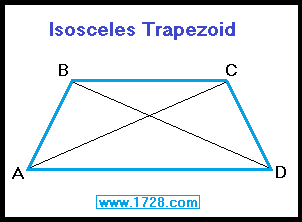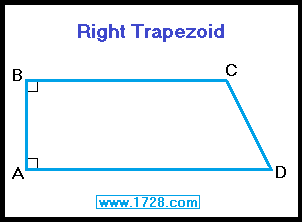The isosceles trapezoid has both legs of equal length.   AB = CD Both diagonals are equal.   AC = BD Lower base angles are equal.   ∠ A = ∠ D Upper base angles are equal.   ∠ B = ∠ C Angles attached to the same leg are supplementary.   ∠ A + ∠ B = 180°   ∠ C + ∠ D = 180° Opposite angles are supplementary.   ∠ A + ∠ C = 180°   ∠ B + ∠ D = 180° The right trapezoid has two right angles. A trapezoid cannot have just one right angle because this prevents any sides from being parallel.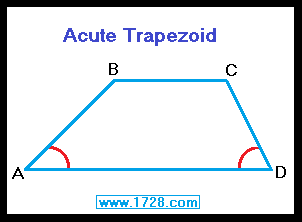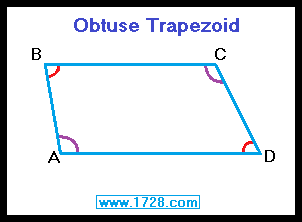The acute trapezoid has two acute angles (A & D) located on each side of the long base (Line AD) and it has two obtuse angles (B & C) on each side of the short base (Line BC). The obtuse trapezoid has two obtuse opposite angles (A & C) and two acute opposite angles (B & D) OR (using the same graphic)       it has one acute angle and one obtuse angle on each base: angles (B & C) and angles (A & D) Significant Figures >>> The default setting is for 5 significant figures but you can change that by inputting another number in the box above. Answers are displayed in scientific notation and for easier readability, numbers between .001 and 1,000 will be displayed in standard format (with the same number of significant figures.) The answers should display properly but there are a few browsers that will show no output whatsoever. If so, enter a zero in the box above. This eliminates all formatting but it is better than seeing no output at all. Return To Geometry Index _____________________ Return To Home Page Copyright © 1999 -     1728 Software Systems